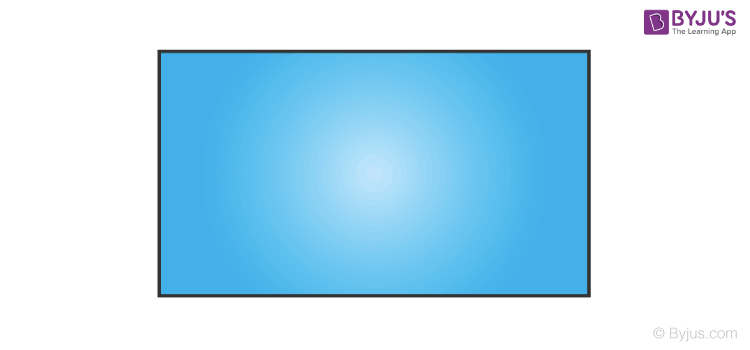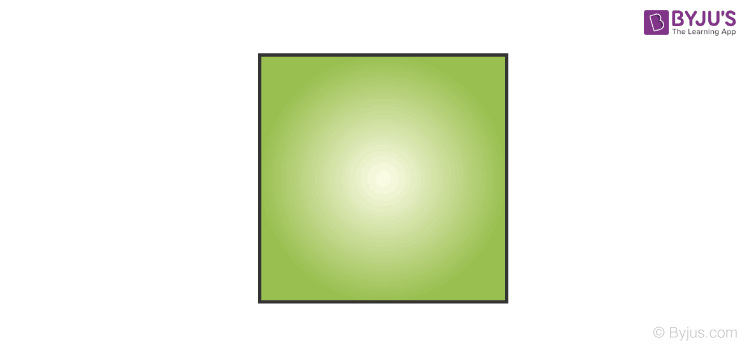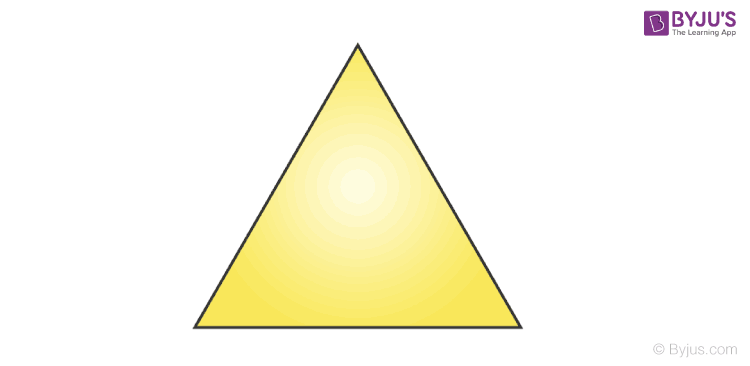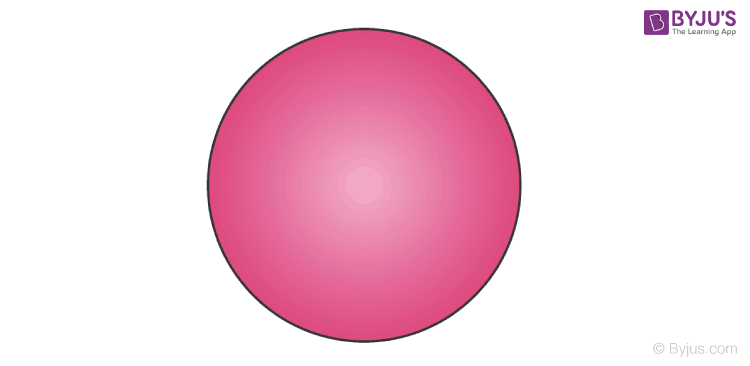Checkout JEE MAINS 2022 Question Paper Analysis : Checkout JEE MAINS 2022 Question Paper Analysis :

# Area and Perimeter

Area and perimeter, in Maths, are the two important properties of two-dimensional shapes. Perimeter defines the distance of the boundary of the shape whereas area explains the region occupied by it.

Area and Perimeter is an important topic in Mathematics, which is used in everyday life. This is applicable to any shape and size whether it is regular or irregular. Every shape has its own area and perimeter formula. You must have learned about different shapes such as triangle, square, rectangle, circle, sphere, etc. The area and perimeter of all shapes are explained here.

## What is Area?

The area is the region bounded by the shape of an object. The space covered by the figure or any two-dimensional geometric shape, in a plane, is the area of the shape. The area of all the shapes depends upon their dimensions and properties. Different shapes have different areas. The area of the square is different from the area of a kite.

If two objects have a similar shape then it’s not necessary that the area covered by them will be equal unless and until the dimensions of both shapes are also equal. Suppose, there are two rectangle boxes, with length as L1 and L2 and breadth as B1 and B2. So the areas of both the rectangular boxes, say A1 and A2 will be equal only if L1=L2 and B1=B2.

## What is Perimeter?

Perimeter of a shape is defined as the total distance around the shape. Basically, perimeter is the length of any shape if it is expanded in a linear form. A perimeter is a total distance that encompasses a shape, in a 2d plane. The perimeter of different shapes can match in length with each other depending upon their dimensions.

For example, if a circle is made of a metal wire of length L, then the same wire we can use to construct a square, whose sides are equal in length.

## What is the Difference Between Area and Perimeter?

Here is the list of differences between area and perimeter:

 Area Perimeter Area is the region occupied by a shape Perimeter is total distance covered by the boundary of a shape Area is measured in square units (m2, cm2, in2, etc.) Perimeter is measured in units (m, cm, in, feet, etc.) Example: Area of rectangular ground is equal to product of its length and breadth. Example: Perimeter of a rectangular ground is equal to sum of all its four boundaries, i.e, 2(length + breadth).

## Area and Perimeter For all Shapes

There are many types of shapes. The most common ones are Square, Triangle, Rectangle, Circle etc. To know the area and perimeter of all these, we need different formulas.

### Perimeter and Area of a Rectangle

A rectangle is a figure/shape with opposite sides equal and all angles equal to 90 degrees. The area of the rectangle is the space covered by it in an XY plane.Perimeter of a Rectangle = 2(a+b) Area of Rectangle = a × b

where a and b are the length and width of the rectangle.

### Perimeter and Area of a Square

A Square is a figure/shape with all four sides equal and all angles equal to 90 degrees. The area of the square is the space occupied by the square in a 2D plane and its perimeter is the distance covered on the outer line.Perimeter of a Square = 4a Area of a Square = a2

where a is the length of the side of the square.

### Perimeter and Area of Triangle

The triangle has three sides. Therefore, the perimeter of any given triangle, whether it is scalene, isosceles or equilateral, will be equal to the sum of the length of all three sides.  And the area of any triangle is the space occupied by it in a plane.Perimeter of a triangle = a + b +c , where a, b and c are the three different sides of the triangle. Area of a triangle = 1/2 b × h; where b is the base and h is the height of the triangle.

### Area and Circumference of Circle

Area of a circle is the region occupied by it in a plane.In case of a circle, the distance of the outer line of the circle is called the circumference.

 Circumference of Circle = 2πr Area of Circle = πr2

## Area and Perimeter Formulas

Here is the list of the area and perimeter for different figures in a tabular form. Students can use this table to solve problems based on the formulas given here.

 Shape Area Perimeter Terms Circle A = π × r2 Circumference = 2πr r = radius of the circle Triangle A = ½ × b × h S = a+b+c b = base h = height a,b and c are the sides of the triangle Square A = a2 P = 4a a = length of side Rectangle A = l × w P = 2(l + w) l = length w = width Parallelogram A = b × h P = 2(a+b) a = side b=base h=vertical height

## Applications of Area and Perimeter

We know that the area is basically the space covered by these shapes and perimeter is the distance around the shape. If you want to paint the walls of your new home, you need to know the area to calculate the quantity of paint required and cost for the same.

For example, to fence the garden in your house, the length required of the material for fencing is the perimeter of the garden. If it’s a square garden with each side as a cm then the perimeter would be 4a cm. The area is the space contained in the shape or the given figure. It is calculated in square units. Suppose you want to fix tiles in your new home, you need to know the area of the floor to know the no. of tiles required to cover the whole floor. In this article, let us have a look at the formula for area and perimeter of some basic shapes with diagrams and examples.

### Area and Perimeter for Grade 4

In Class 4, we will come across the area and perimeter formulas for square and rectangle shapes. Here, the sides of rectangle are measured in inches or feet or yard. Let us see an example.

Example: Find the area of a rectangle with length and width equal to 7ft and 5ft, respectively.
Solution: Given,
Length = 7ft & Width = 5ft
Area of rectangle = Length x Width
= 7ft x 5ft
= 35 ft2

## Related Articles

 Area Related Articles Perimeter Related articles Area of a Circle Circumference of a Circle Area of Triangle Perimeter of Triangle Area of Rectangle Perimeter of Rectangle Area Of A Square Perimeter of Square Area of Parallelogram Perimeter of a Parallelogram

## Solved Examples on Area and Perimeter

Here are some solved examples based on the formulas of the area as well as perimeter of different shapes.

Example 1:

If the radius of a circle is 21cm. Find its area and circumference.

Solution:

Therefore, Area = π × r2

A = 22/7 × 21 × 21

A = 1386 sq.cm.

Circumference, C = 2πr

C = 2 x 22/7 x 21 = 132 cm

Example 2:

If the length of the side of a square is 11cm. Then find its area and also find the total length of its boundary.

Solution:

Given, length of the side, a = 11 cm

Area = a= 11= 121 sq.cm

Total length of its boundary, Perimeter = 4a = 4 x 11 = 44 sq.cm.

Example 3:

The length of rectangular field is 12m and width is 10m. Find the area of the field along with its perimeter.

Solution:

Given, Length = 12m

Width = 10m

Therefore, Area = length x width = 12 x 10 = 120 sq.m.

Perimeter = 2 (length + width) = 2 x (12 + 10) = 2 x 22 = 44 m.

## Practice Problems (Worksheets)

Solve the questions here based on area and perimeter of different shapes.

1. If a square has area 625 sq.m., then find its perimeter.
2. The length and width of a rectangle are 11.5 cm and 8.8 cm, respectively. Find its area and perimeter.
3. If the height of a triangle is 19cm and its base length is 12cm. Find the area.
4. The perimeter of an equilateral triangle is 21cm. Find its area.
5. A parallelogram has base length of 16cm and the distance between the base and its opposite side is 7.5cm. Find its area.

Register at BYJU’S – The Learning App to learn other mathematical concepts and download its app to get personalized videos.

## Frequently Asked Questions – FAQs

### What is the difference between area and perimeter?

The area is the region covered by shape or figure whereas perimeter is the distance covered by outer boundary of the shape.
The unit of area is given by square unit or unit2 and unit of perimeter is same as the unit.

### What is the formula for perimeter?

The perimeter of any polygon is equal to the sum of its sides.
Perimeter = Sum of all sides

### What is the area and perimeter of a circle?

A circle is a curved shape and its area and perimeter are given by its radius.
Area of circle is πr2
Perimeter or circumference of circle is 2πr.

### What is the perimeter and area example?

If a square has side length of 2cm then,
Area of square = side2 = 22 = 4cm2
Perimeter of square = sum of all sides = 2+2+2+2 = 8

### What is the formula for area of rectangle?

The area of rectangle is equal to the product of its length and breadth.